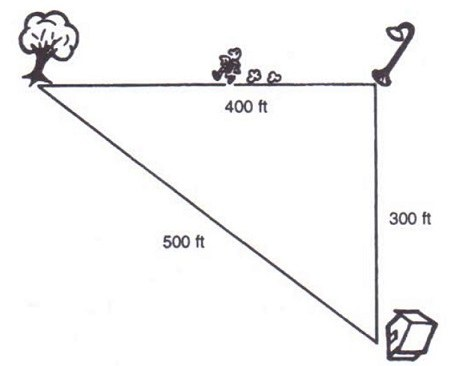# Velocity

Velocity tells us more about things that we need to know about motion
👁 154
statistics
Questions #: 11
Time: 5 minutes
Pass Score: 80.0%
Style
Mode

POINTS (1)

#### Identify the following two descriptions below as speed or velocity

1. 20 meters/second
2. 20 meters/second northeast
drag and drop the selected option to the right place or type it instead
velocity
speed
POINTS (1)

#### The defining equation for velocity is

1. v = d / t
2. v = d * t
3. v = t / d
4. v = (t * d)2
POINTS (1)

POINTS (1)

v = d / t

POINTS (1)

#### Symbol d in velocity equation

is called object's displacement and defined as the _______-line distance from object's starting position to its final position, including the direction from start to finish

v = dt

POINTS (1)

#### Mark the vector quantities

v = d / t

v is

d is

t is

drag and drop the selected option to the right place or type it instead
Vector
Not Vector
Not Applicable
POINTS (1)

POINTS (1)

#### Identify each of the following quantities as vector or scalar

1. velocity
2. speed
3. time
4. displacement
drag and drop the selected option to the right place or type it instead
vector
scalar
POINTS (1)

POINTS (1)

#### Jogger Example

Suppose a jogger leaves her house and jogs north 300 meters to the streetlamp on the corner.  She turns west, jogs another 300 meters, and gets the oak tree 2 minutes after leaving home1. Which of the three distances given in the figure is the displacement d?
2. What is the jogger's average velocity?
3. What is the total distance (not displacement) travelled?
4. What is the average speed (not velocity) during the 2 minutes?

drag and drop the selected option to the right place or type it instead
350 meters/min
500 meters
700 meters
250 meters/min in a direction between north and west
POINTS (1)